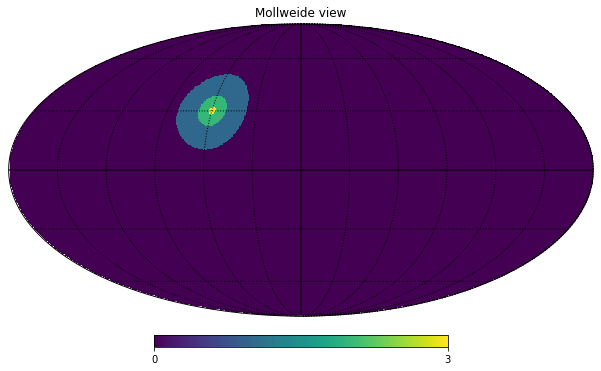# Example of the healpy query disc function

python
healpy
cosmology
Published

October 2, 2020

import healpy as hp
import numpy as np

lonlat=True switches ang2vec from requiring colatitude $$\theta$$ and longitude $$\phi$$ in radians to longitude and latitude in degrees (notice that also the order changes)

# in degrees
lon = 60
lat = 30
vec = hp.ang2vec(lon, lat, lonlat=True)
nside = 256
tiny_disc = hp.query_disc(nside, vec, radius=np.radians(2))

query_disc returns a list of pixels, by default in RING ordering, let’s check their length:

list(map(len, [large_disc, small_disc, tiny_disc]))
[23715, 3829, 237]

## Create a map and plot it in Mollweide projection

m = np.zeros(hp.nside2npix(nside))
m[large_disc] = 1
m[small_disc] = 2
m[tiny_disc] = 3
hp.mollview(m)
hp.graticule()
0.0 180.0 -180.0 180.0
The interval between parallels is 30 deg -0.00'.
The interval between meridians is 30 deg -0.00'.